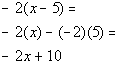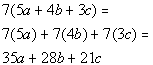# Beginning Algebra Tutorial 8

Beginning Algebra
Tutorial 8: Properties of Real NumbersAnswer/Discussion to 1a

xy

Using the commutative property of multiplication (where changing the order of a product does not change the value of it), we get

xy = yxAnswer/Discussion to 1b

.1 + 3x

Using the commutative property of addition (where changing the order of a sum does not change the value of it), we get

.1 + 3x = 3x + .1Answer/Discussion to 2a

(a + b) + 1.5

Using the associative property of addition (where changing the grouping of a sum does not change the value of it), we get

(a + b) + 1.5 = a + (b + 1.5)Answer/Discussion to 2b

5(xy)

Using the associative property of multiplication (where changing the grouping of a product does not change the value of it), we get

5(xy) = (5x)yAnswer/Discussion to 3a

-2(x - 5)*Distribute -2 to EVERY term
*MultiplyAnswer/Discussion to 3b

7(5a + 4b + 3c)*Distribute 7 to EVERY term
*MultiplyAnswer/Discussion to 4a

-7

The opposite of -7 is 7, since -7 + 7 = 0.

The reciprocal of -7 is -1/7, since -7(-1/7) = 1.Answer/Discussion to 4b

3/5

The opposite of 3/5 is -3/5, since 3/5 + (-3/5) = 0.

The reciprocal of 3/5 is 5/3, since (3/5)(5/3) = 1.

Last revised on July 24, 2011 by Kim Seward.